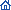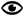Main / About us / University structure / Departments / Academic Affairs Department / Registration office / GPA CalculatorAccording to the results of examinations for the course based on the results the summer semester grade point average is determined by the student (GPA), calculated by dividing the sum of the products of credits and the grade of the discipline (in numerical terms) to total loans for the current academic period.

GPA is recorded in the student transcript. GPA is calculated by the formula:

GPA = Its1 * K1 + K1 + Its2 * ........ * Itsn Kn

K1 + K2 + ............... Kn.

Its1 ....... the resulting estimates of the disciplines in the digital equivalent;

K1 .................... volumes of subjects studied in the credits;

n - the number of subjects studied during the entire training period passed.

Example of calculating the GPA:

 Discipline Number Credit Score from letter system Digital equivalent scores informatics 3 A 4.0 sociology 2 B 3.0 philosophy 3 F 0.0

Informatics  4.0 x 3 = 12.0

Sociology of 3.0 x 2 = 6.0

Philosophy of 0.0 x 3 = 0.0

The sum of products = 12 + 6 + 0 = 18.0

The total number of credits = 3 +2 +3 = 8

GPA = sum of the products / total loans = 18.0 / 8 = 2.25

To proceed to the next course is not enough to pass all the exams to the positive evaluation; you need to dial the minimum transfer GPA score

On the 2013/2014 academic year was approved following a transfer score GPA:

With the first course on the second - 1,80;

A second course in the third - 2.00;

A third course on the 4th - 2.20.

The student is not secured the minimum conversion score remains at a second course of study.

Примечание: Дробная часть цифрового эквивалента отделяется от целой - точкой.

Discipline The number of credits The digital equivalent

Result:827